# RD Sharma Solutions for Class 8 Maths Chapter 4 - Cubes and Cube Roots Exercise 4.1

In Exercise 4.1 of RD Sharma Class 8 Maths Chapter 4 Cubes and Cube Roots, we shall discuss problems based on the cubes of natural numbers and perfect cube numbers along with their properties, finding cube of a two-digit number by column method. Students can refer and download RD Sharma Class 8 Solutions Maths from the links provided below. RD Sharma Solutions provides ample questions along with their solutions, shortcut techniques and detailed explanations are provided by our expert tutors, which help students score well in their examination.

## Download PDF of RD Sharma Solutions for Class 8 Maths Exercise 4.1 Chapter 4 Cubes and Cube Roots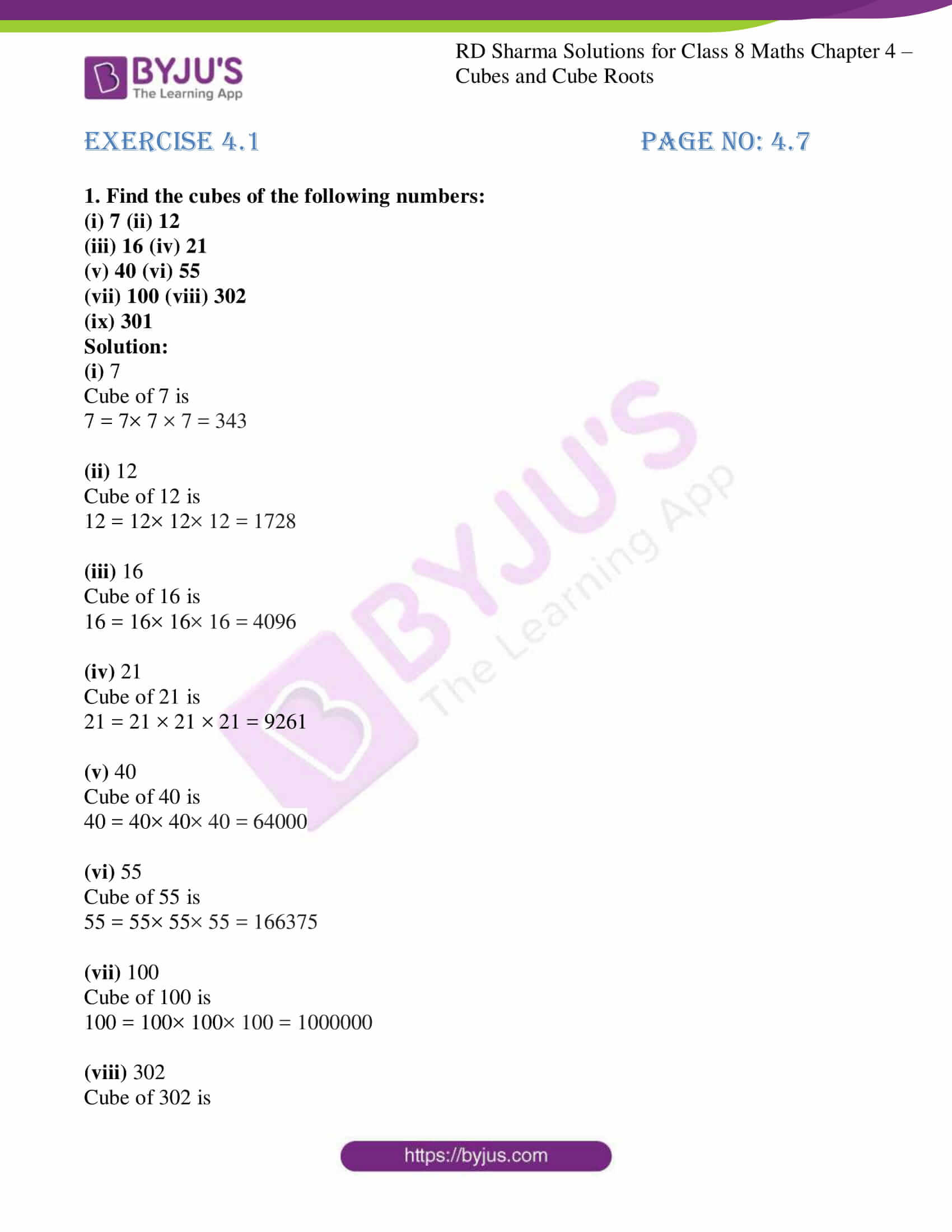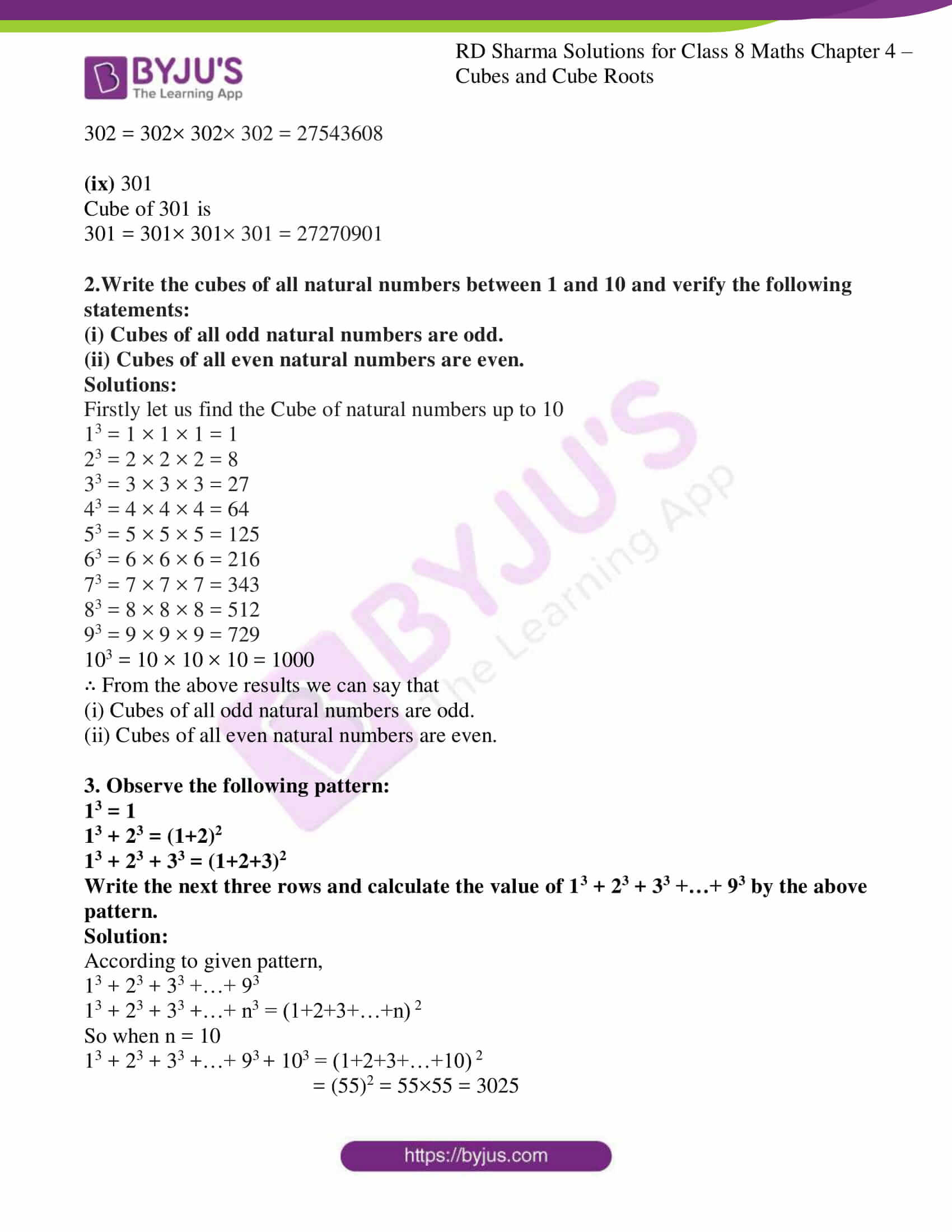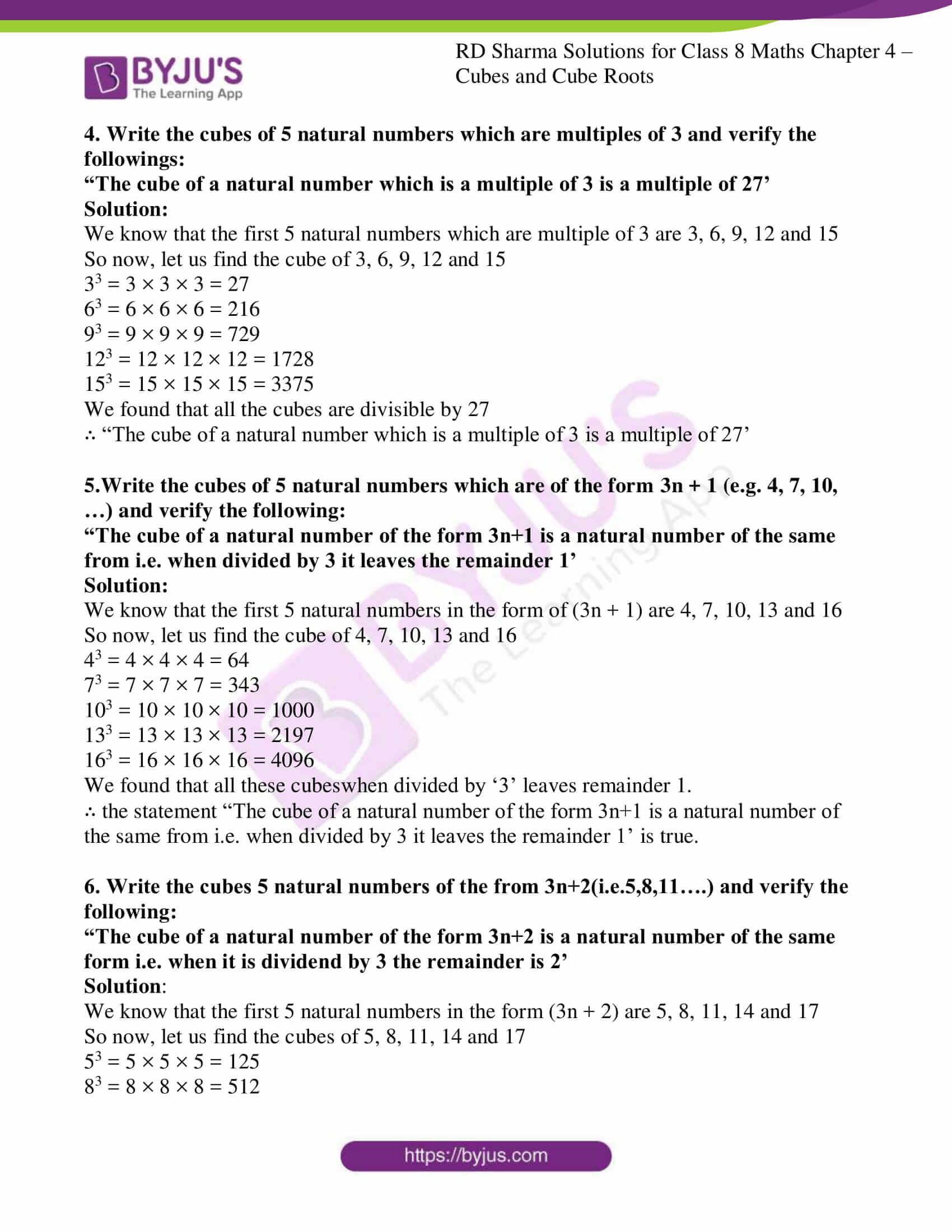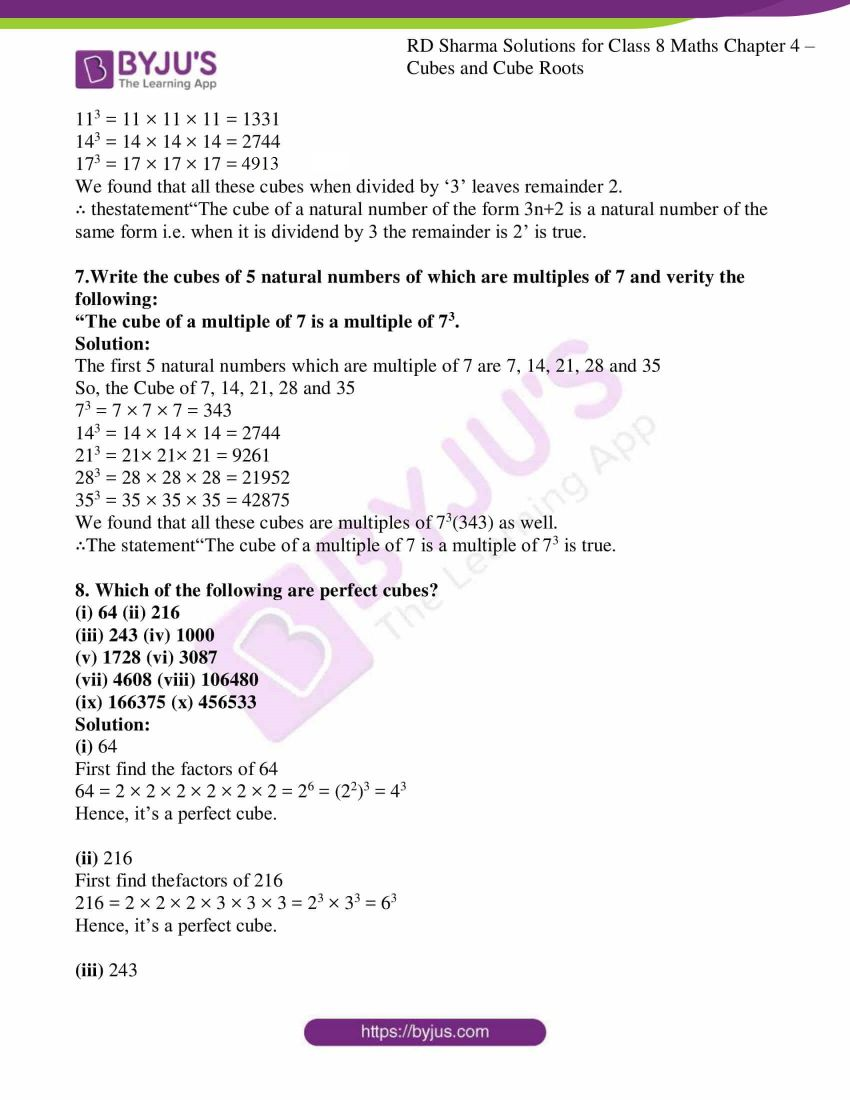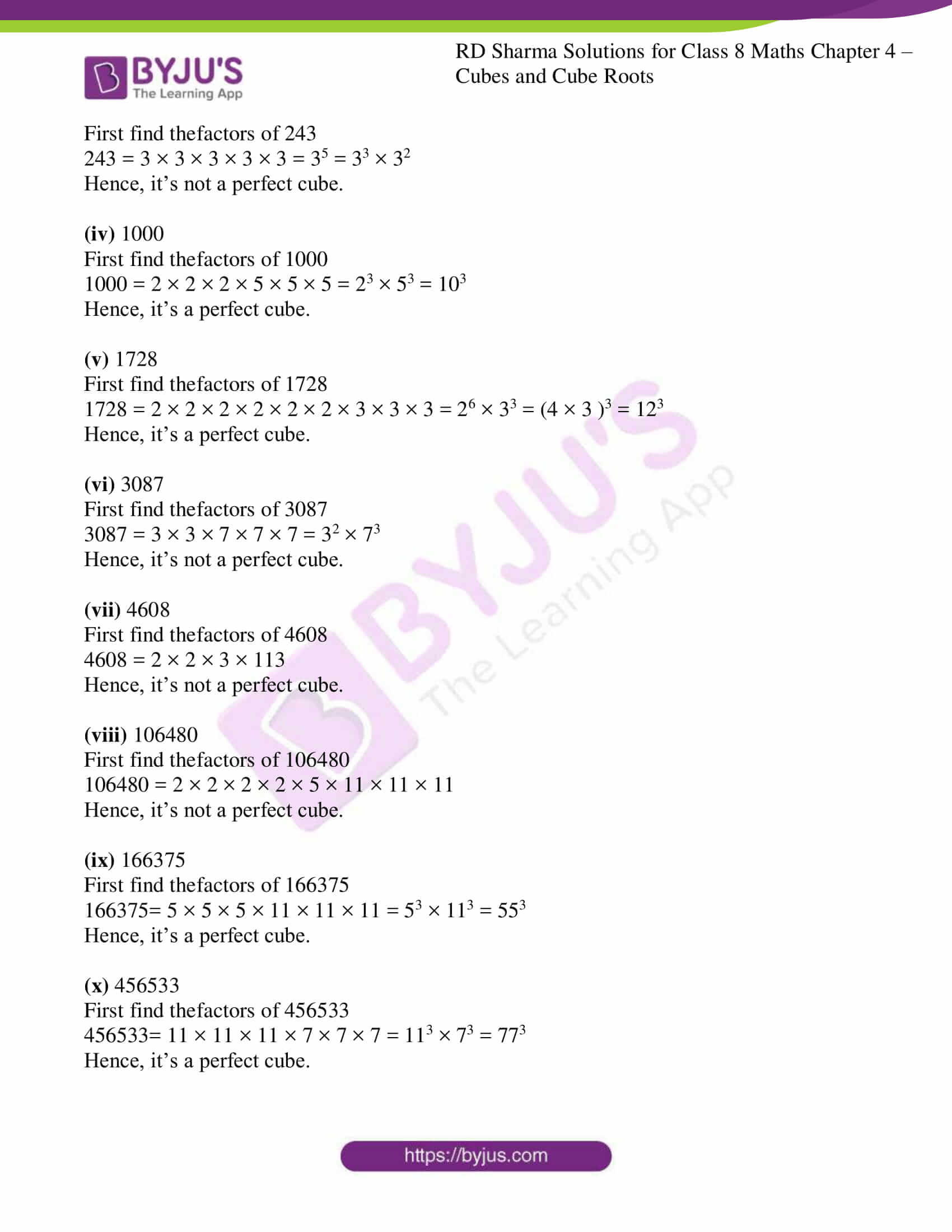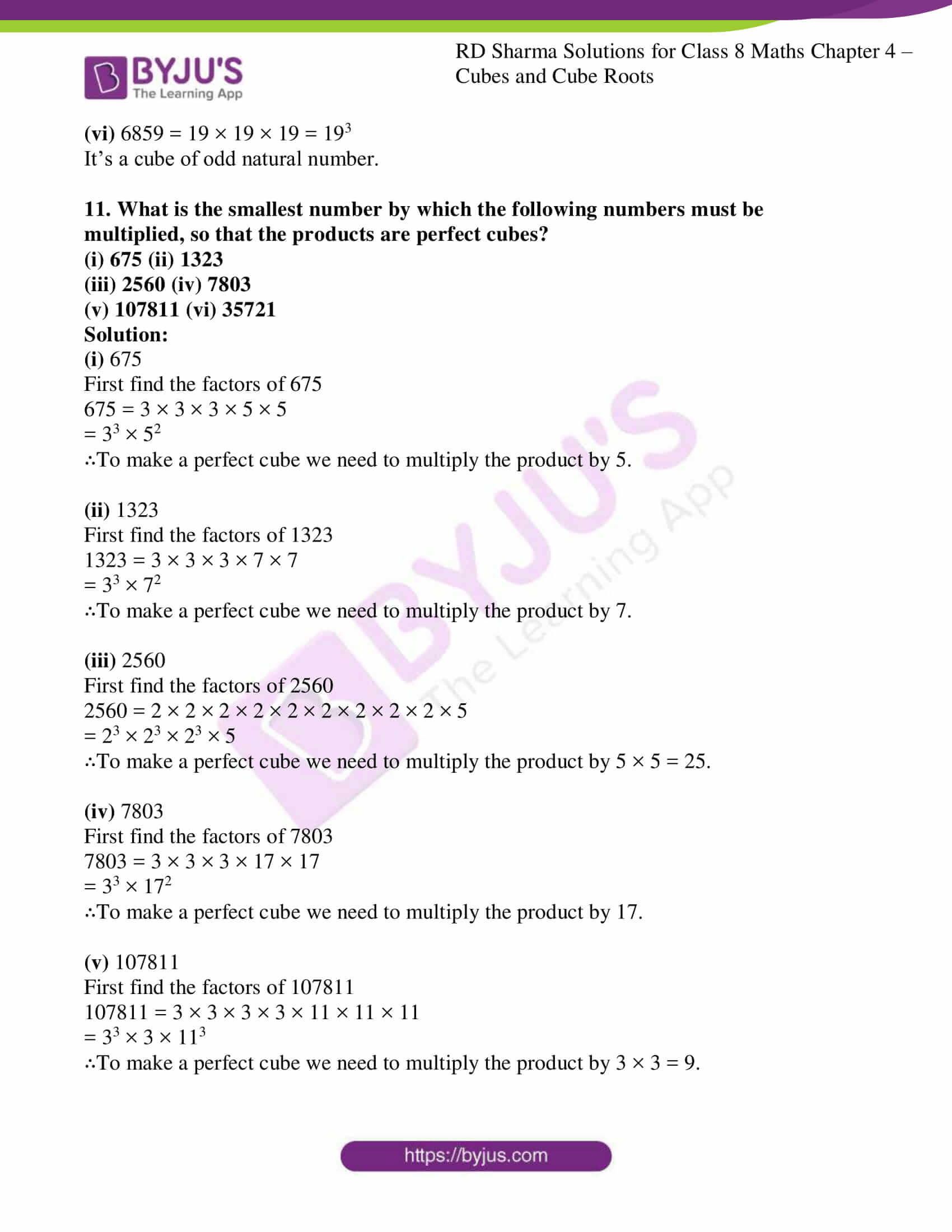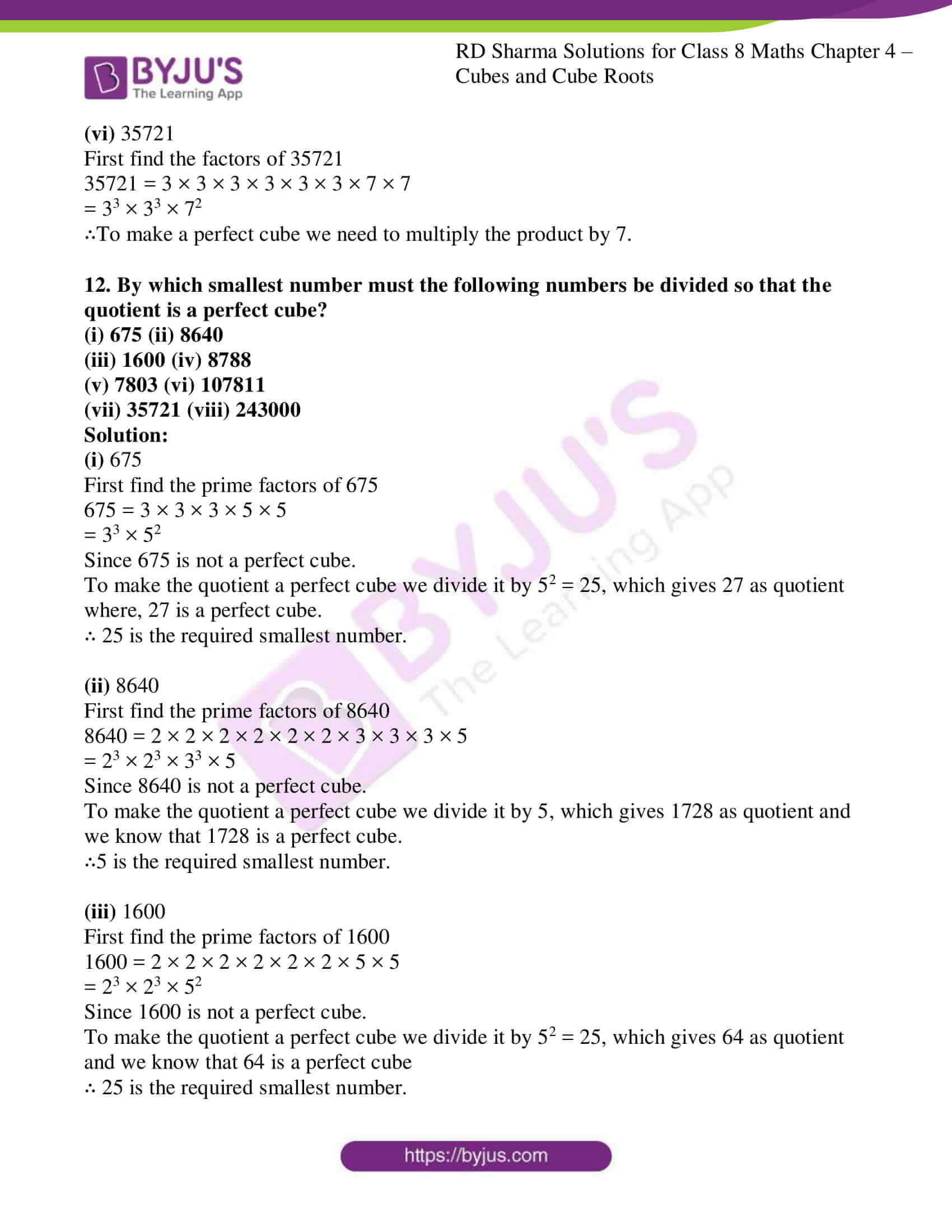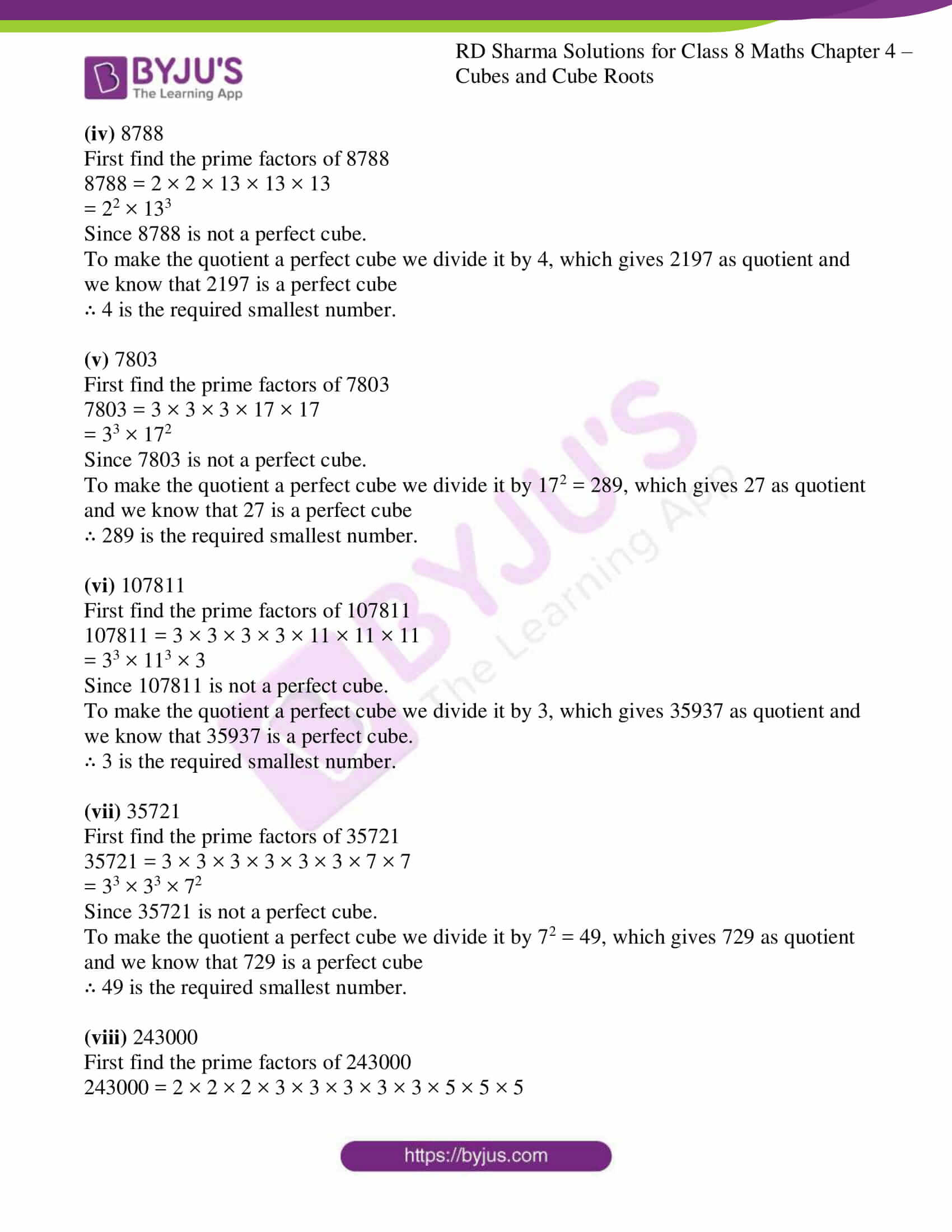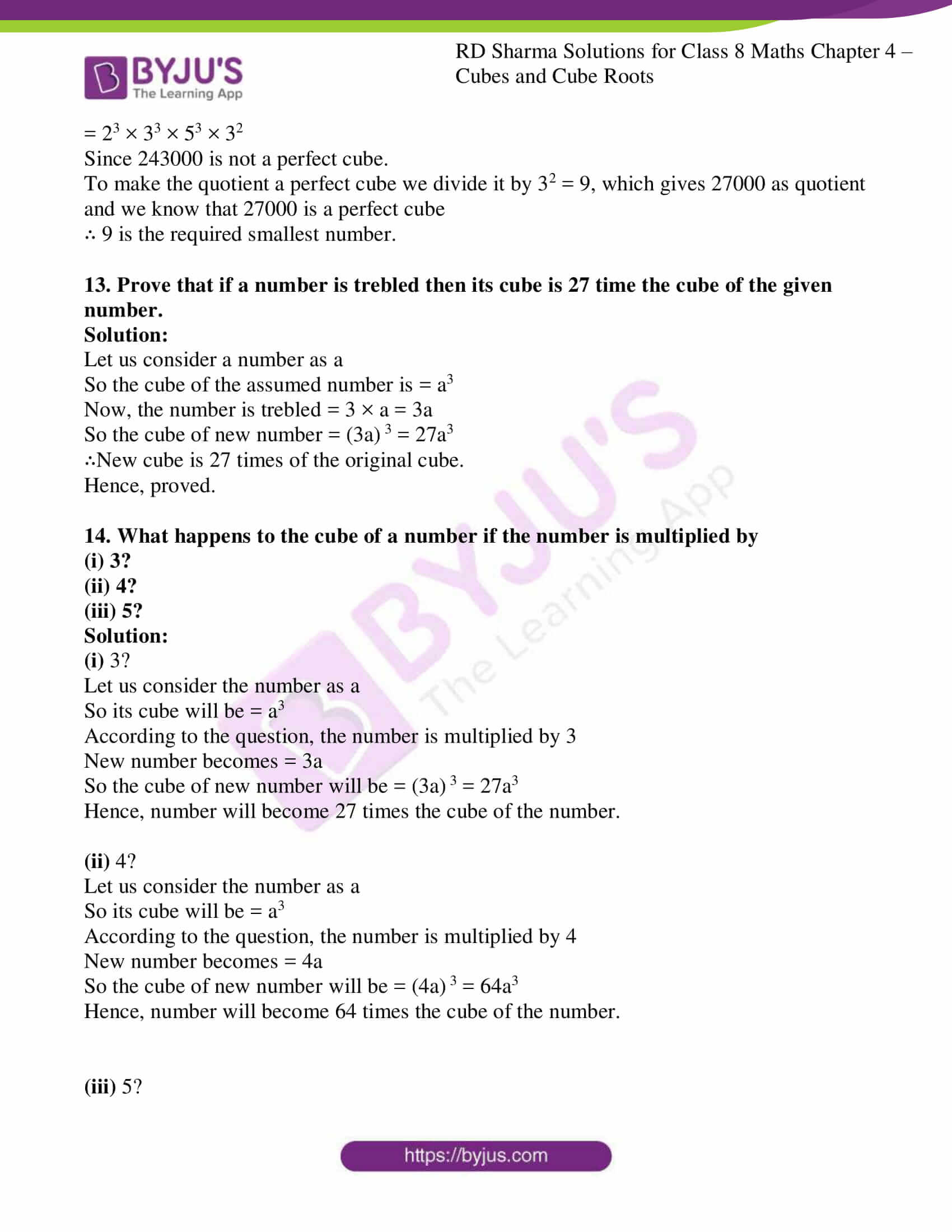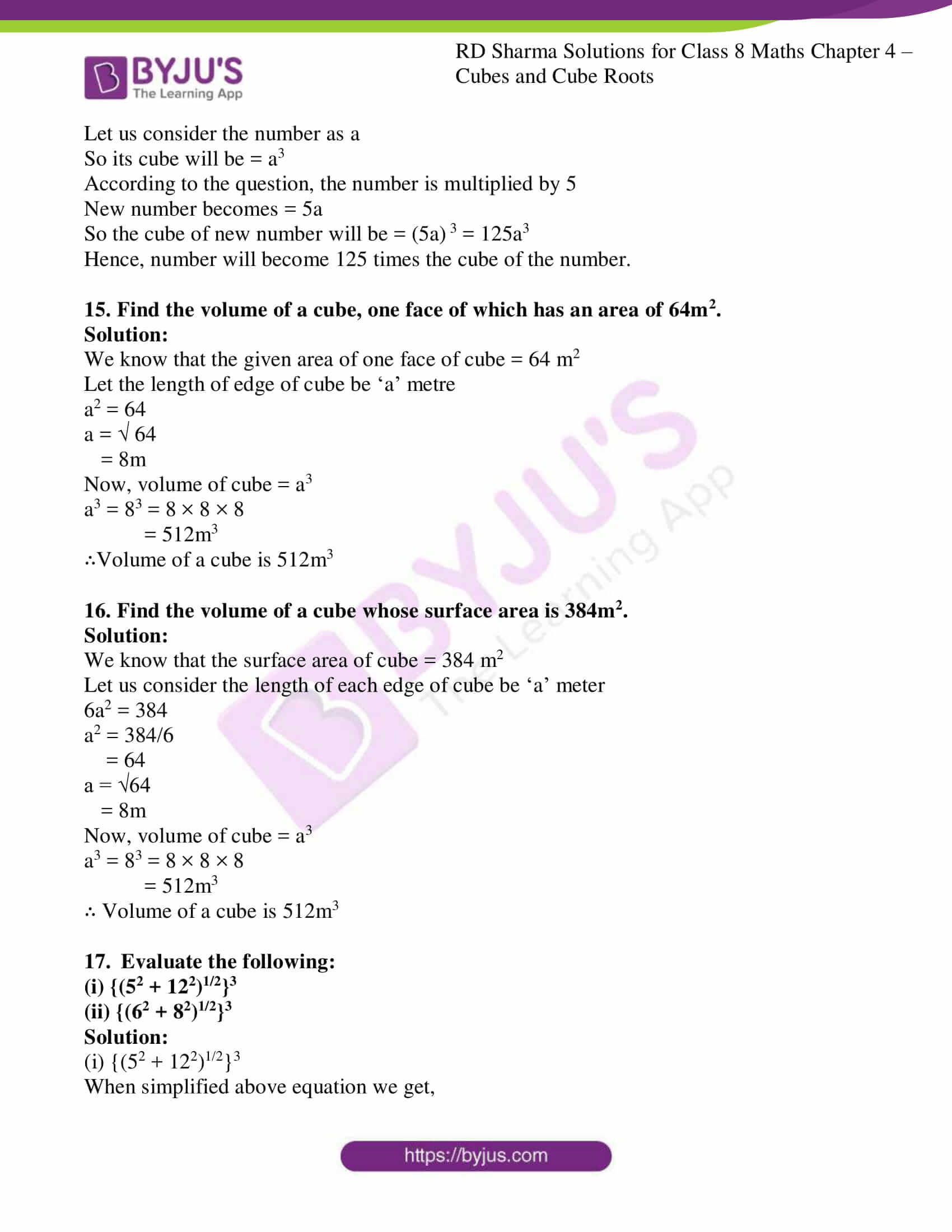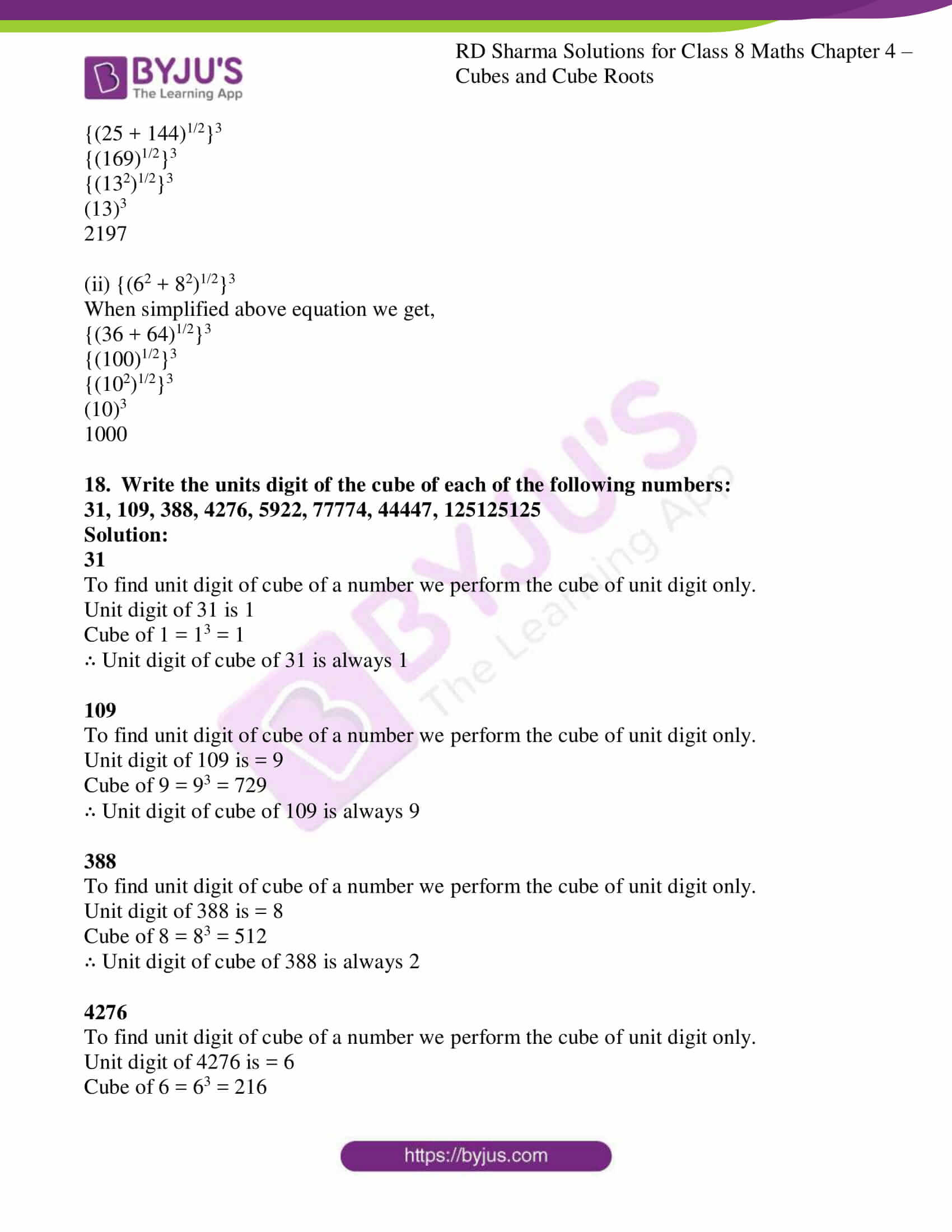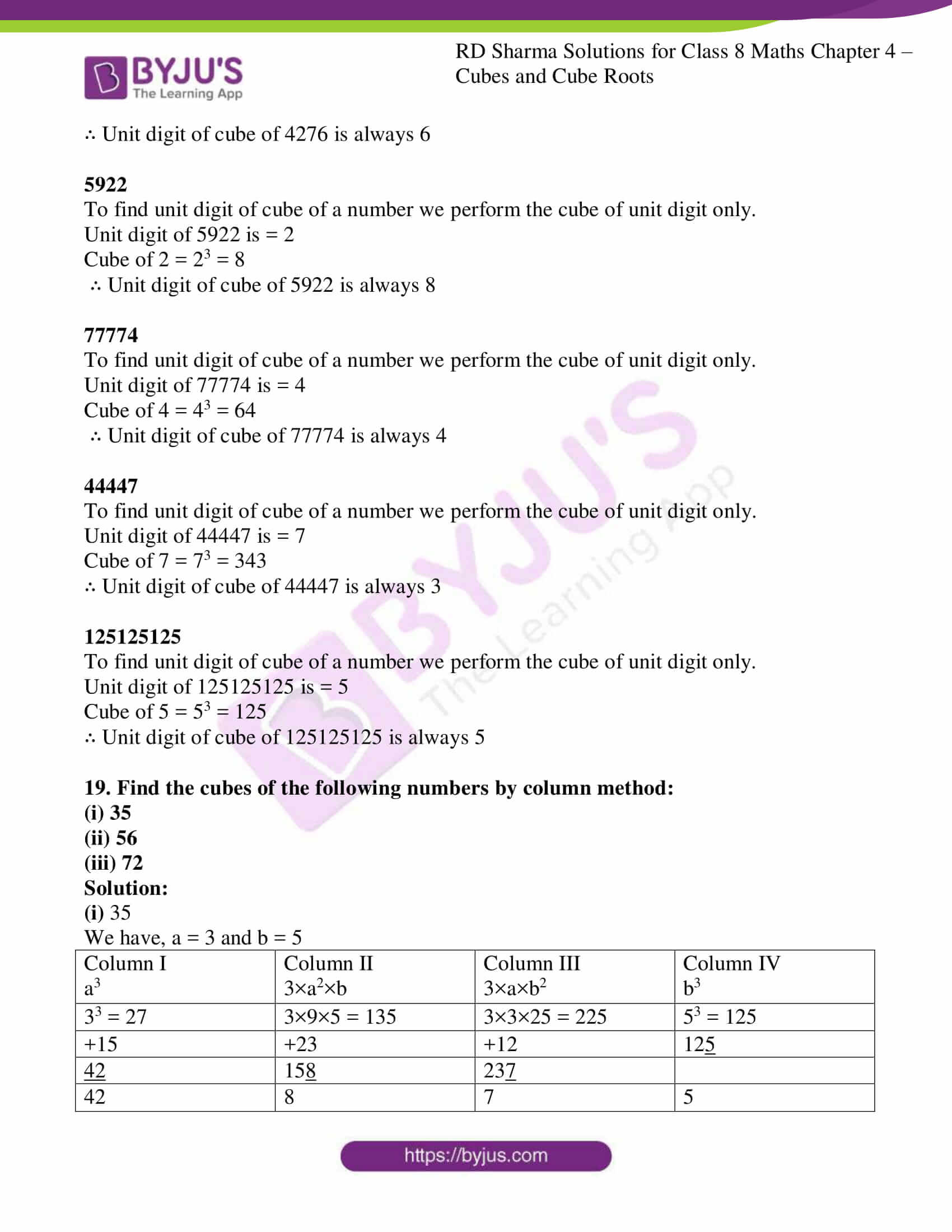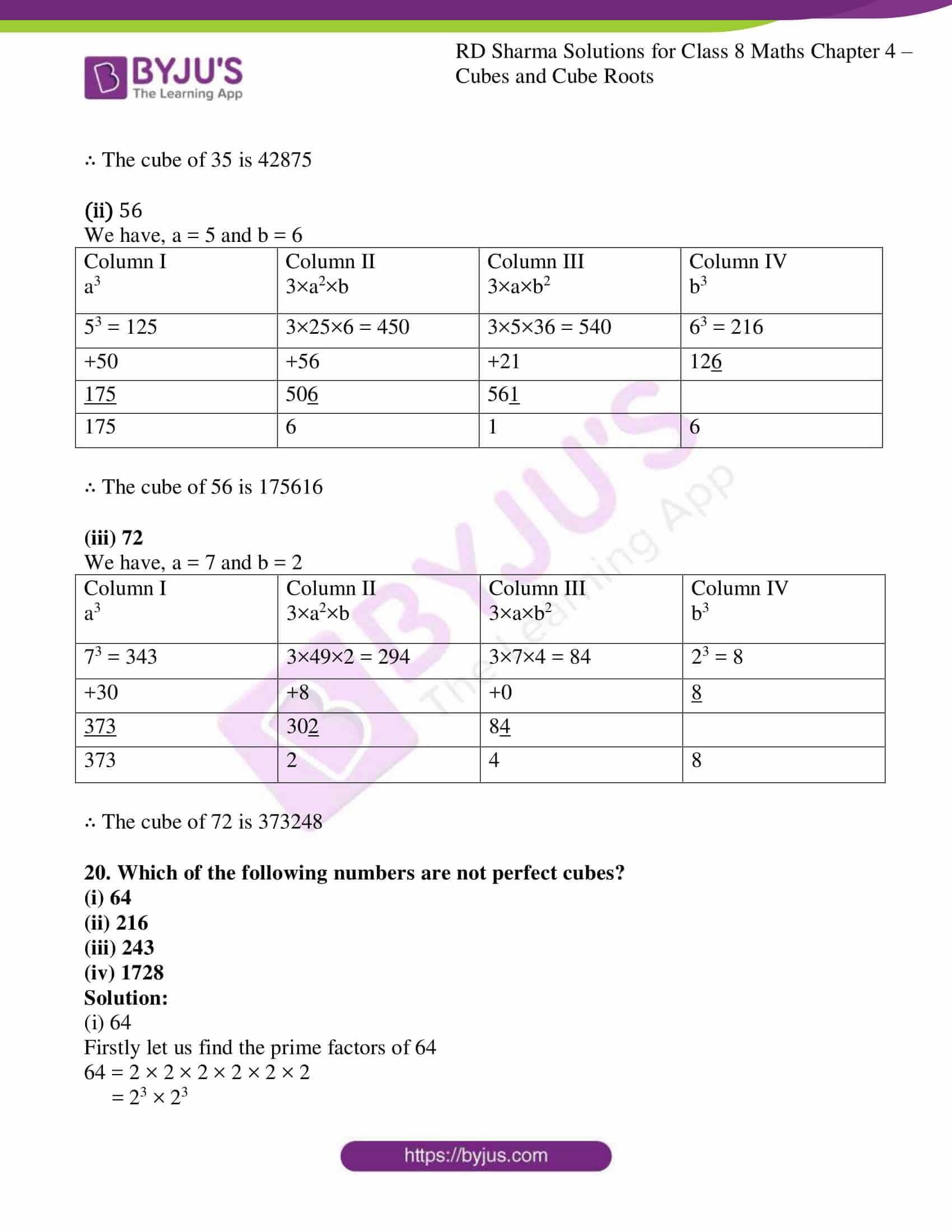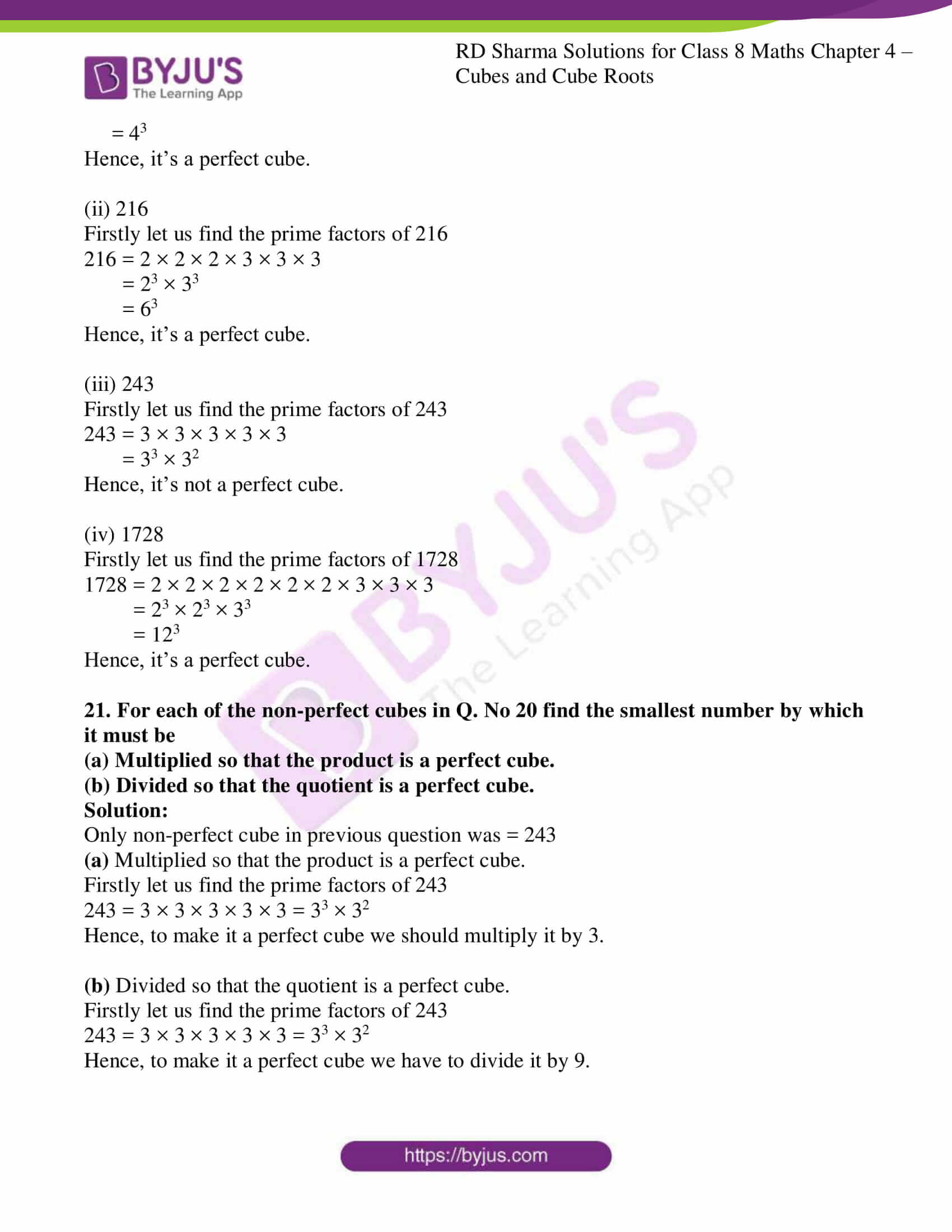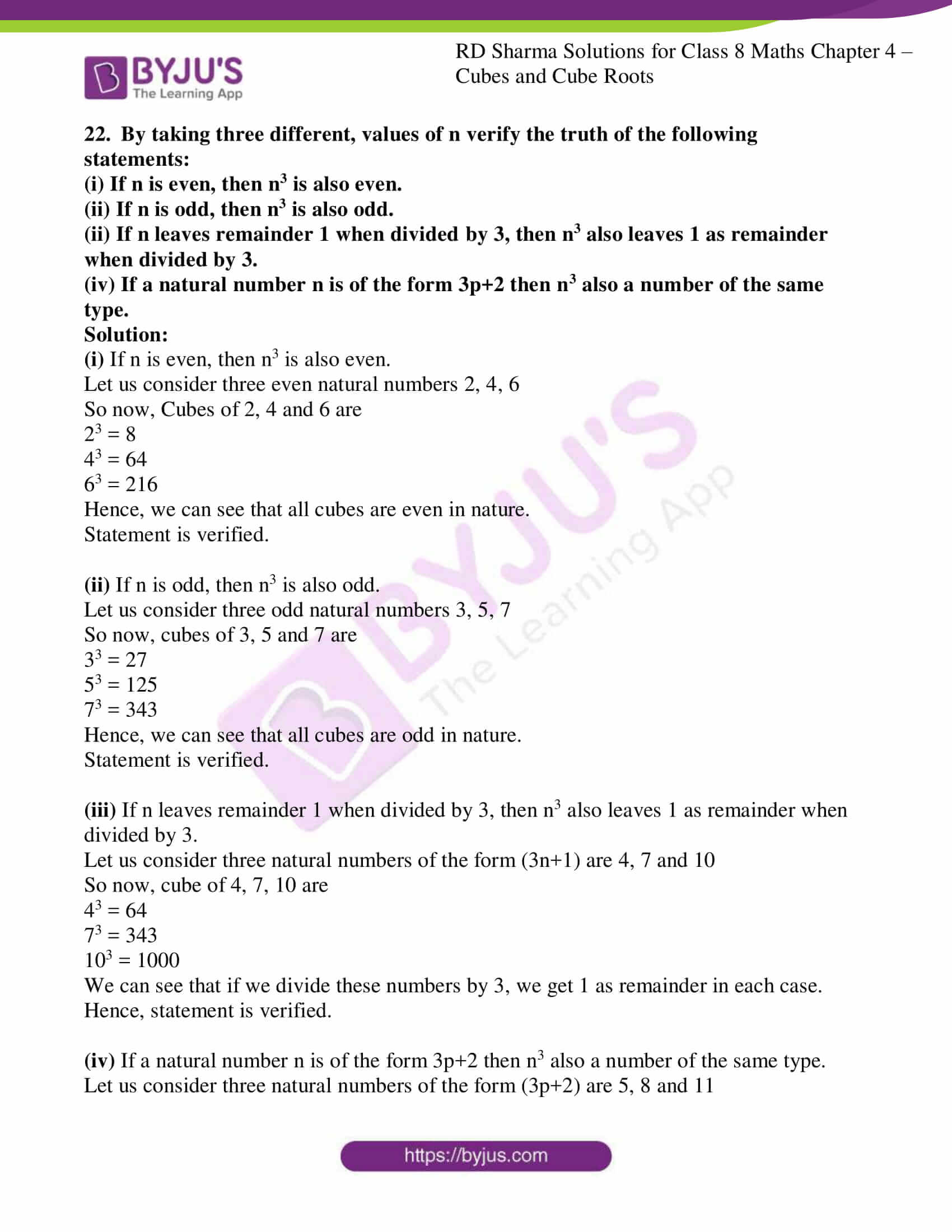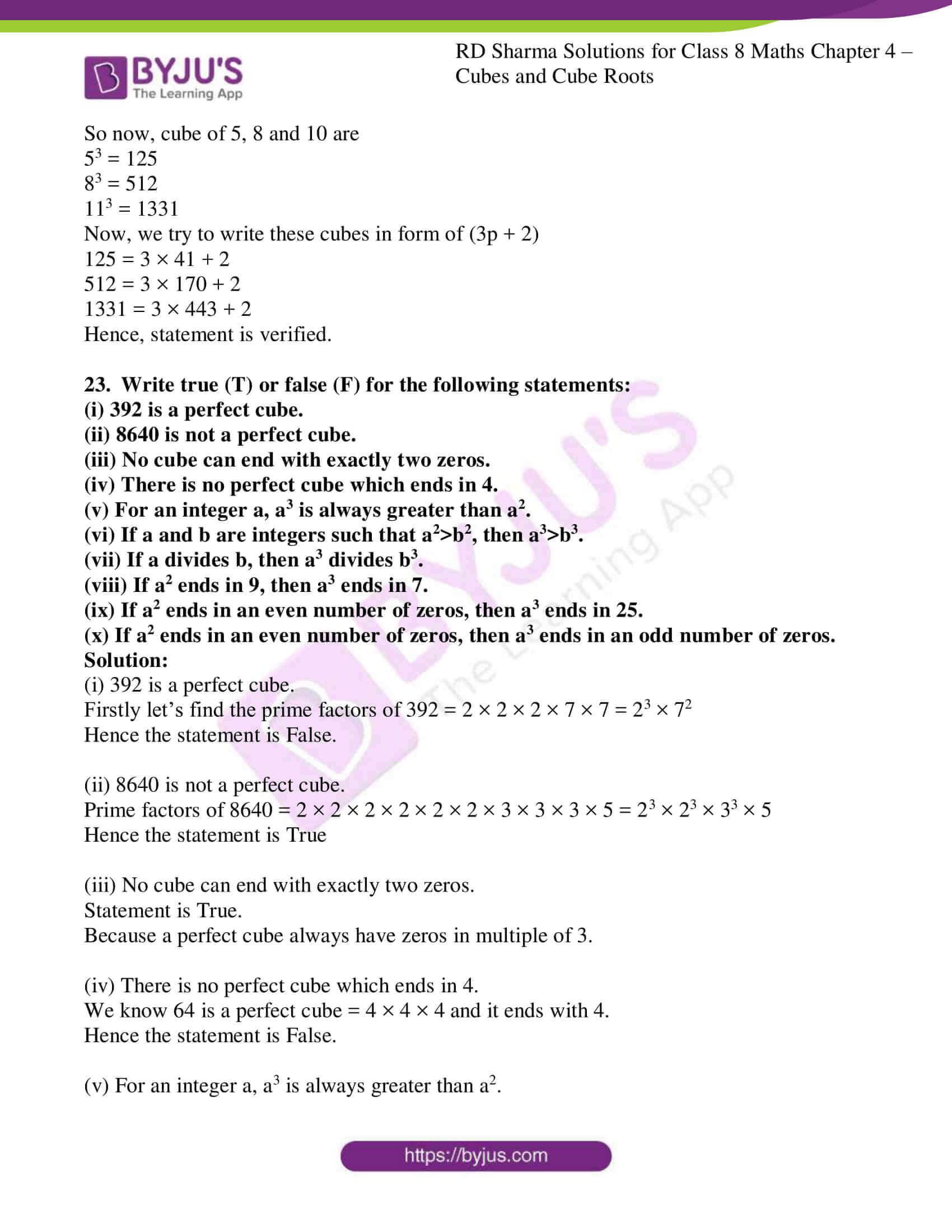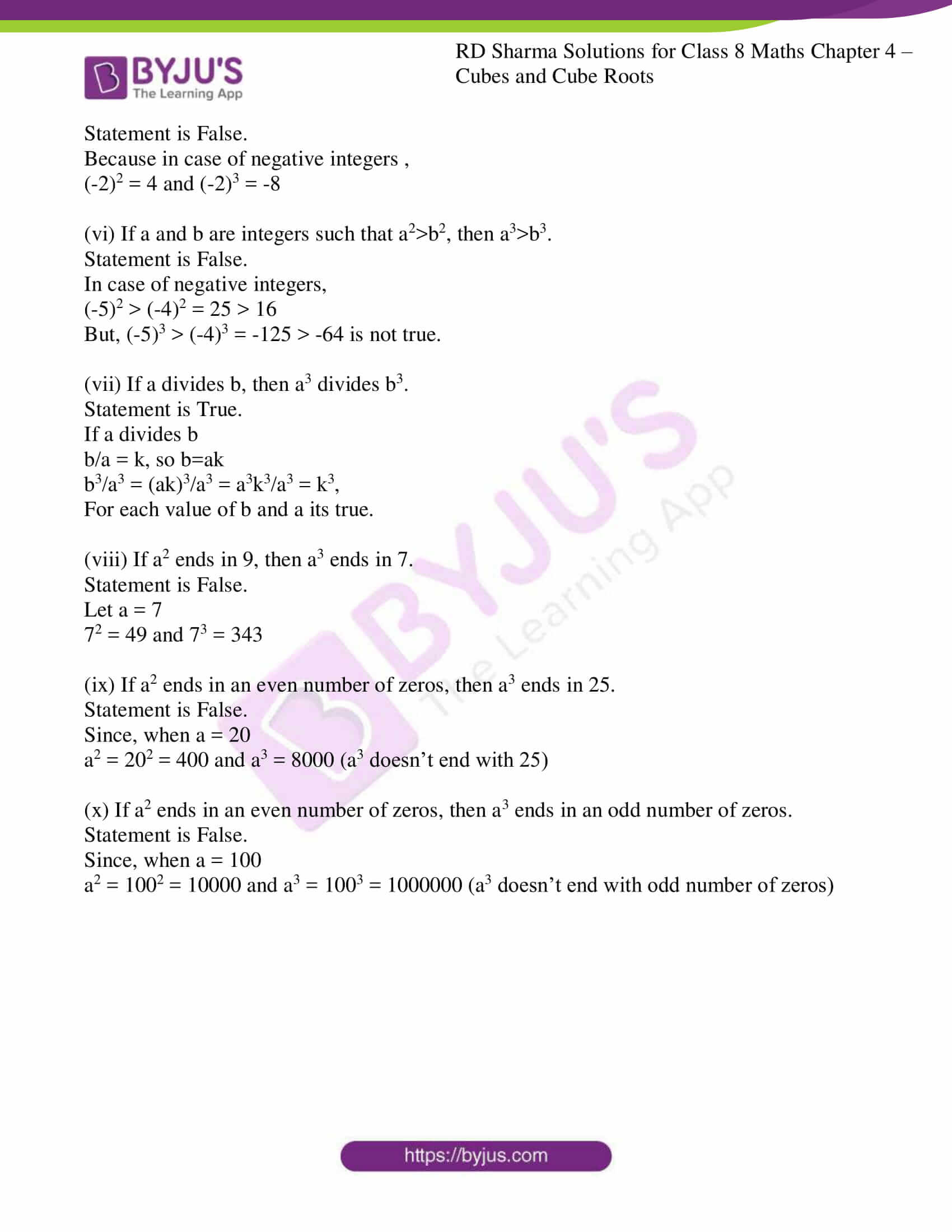### Access Answers to RD Sharma Solutions for Class 8 Maths Exercise 4.1 Chapter 4 Cubes and Cube Roots

1. Find the cubes of the following numbers:
(i) 7 (ii) 12

(iii) 16 (iv) 21

(v) 40 (vi) 55

(vii) 100 (viii) 302

(ix) 301

Solution:

(i) 7

Cube of 7 is

7 = 7× 7 × 7 = 343

(ii) 12

Cube of 12 is

12 = 12× 12× 12 = 1728

(iii) 16

Cube of 16 is

16 = 16× 16× 16 = 4096

(iv) 21

Cube of 21 is

21 = 21 × 21 × 21 = 9261

(v) 40

Cube of 40 is

40 = 40× 40× 40 = 64000

(vi) 55

Cube of 55 is

55 = 55× 55× 55 = 166375

(vii) 100

Cube of 100 is

100 = 100× 100× 100 = 1000000

(viii) 302

Cube of 302 is

302 = 302× 302× 302 = 27543608

(ix) 301

Cube of 301 is

301 = 301× 301× 301 = 27270901

2.Write the cubes of all natural numbers between 1 and 10 and verify the following statements:
(i) Cubes of all odd natural numbers are odd.
(ii) Cubes of all even natural numbers are even.

Solutions:

Firstly let us find the Cube of natural numbers up to 10

13 = 1 × 1 × 1 = 1

23 = 2 × 2 × 2 = 8

33 = 3 × 3 × 3 = 27

43 = 4 × 4 × 4 = 64

53 = 5 × 5 × 5 = 125

63 = 6 × 6 × 6 = 216

73 = 7 × 7 × 7 = 343

83 = 8 × 8 × 8 = 512

93 = 9 × 9 × 9 = 729

103 = 10 × 10 × 10 = 1000

∴ From the above results we can say that

(i) Cubes of all odd natural numbers are odd.

(ii) Cubes of all even natural numbers are even.

3. Observe the following pattern:
13 = 1

13 + 23 = (1+2)2

13 + 23 + 33 = (1+2+3)2
Write the next three rows and calculate the value of 13 + 23 + 33 +…+ 93 by the above pattern.

Solution:

According to given pattern,

13 + 23 + 33 +…+ 93

13 + 23 + 33 +…+ n3 = (1+2+3+…+n) 2

So when n = 10

13 + 23 + 33 +…+ 93 + 103 = (1+2+3+…+10) 2

= (55)2 = 55×55 = 3025

4. Write the cubes of 5 natural numbers which are multiples of 3 and verify the followings:
“The cube of a natural number which is a multiple of 3 is a multiple of 27’

Solution:

We know that the first 5 natural numbers which are multiple of 3 are 3, 6, 9, 12 and 15

So now, let us find the cube of 3, 6, 9, 12 and 15

33 = 3 × 3 × 3 = 27

63 = 6 × 6 × 6 = 216

93 = 9 × 9 × 9 = 729

123 = 12 × 12 × 12 = 1728

153 = 15 × 15 × 15 = 3375

We found that all the cubes are divisible by 27

∴ “The cube of a natural number which is a multiple of 3 is a multiple of 27’

5.Write the cubes of 5 natural numbers which are of the form 3n + 1 (e.g. 4, 7, 10, …) and verify the following:
“The cube of a natural number of the form 3n+1 is a natural number of the same from i.e. when divided by 3 it leaves the remainder 1’

Solution:

We know that the first 5 natural numbers in the form of (3n + 1) are 4, 7, 10, 13 and 16

So now, let us find the cube of 4, 7, 10, 13 and 16

43 = 4 × 4 × 4 = 64

73 = 7 × 7 × 7 = 343

103 = 10 × 10 × 10 = 1000

133 = 13 × 13 × 13 = 2197

163 = 16 × 16 × 16 = 4096

We found that all these cubes when divided by ‘3’ leaves remainder 1.

∴ the statement “The cube of a natural number of the form 3n+1 is a natural number of the same from i.e. when divided by 3 it leaves the remainder 1’ is true.

6. Write the cubes 5 natural numbers of the from 3n+2(i.e.5,8,11….) and verify the following:
“The cube of a natural number of the form 3n+2 is a natural number of the same form i.e. when it is dividend by 3 the remainder is 2’

Solution:

We know that the first 5 natural numbers in the form (3n + 2) are 5, 8, 11, 14 and 17

So now, let us find the cubes of 5, 8, 11, 14 and 17

53 = 5 × 5 × 5 = 125

83 = 8 × 8 × 8 = 512

113 = 11 × 11 × 11 = 1331

143 = 14 × 14 × 14 = 2744

173 = 17 × 17 × 17 = 4913

We found that all these cubes when divided by ‘3’ leaves remainder 2.

∴ the statement“The cube of a natural number of the form 3n+2 is a natural number of the same form i.e. when it is dividend by 3 the remainder is 2’ is true.

7.Write the cubes of 5 natural numbers of which are multiples of 7 and verity the following:
“The cube of a multiple of 7 is a multiple of 73.

Solution:

The first 5 natural numbers which are multiple of 7 are 7, 14, 21, 28 and 35

So, the Cube of 7, 14, 21, 28 and 35

73 = 7 × 7 × 7 = 343

143 = 14 × 14 × 14 = 2744

213 = 21× 21× 21 = 9261

283 = 28 × 28 × 28 = 21952

353 = 35 × 35 × 35 = 42875

We found that all these cubes are multiples of 73(343) as well.

∴The statement“The cube of a multiple of 7 is a multiple of 73 is true.

8. Which of the following are perfect cubes?
(i) 64 (ii) 216
(iii) 243 (iv) 1000
(v) 1728 (vi) 3087
(vii) 4608 (viii) 106480
(ix) 166375 (x) 456533

Solution:

(i) 64

First find the factors of 64

64 = 2 × 2 × 2 × 2 × 2 × 2 = 26 = (22)3 = 43

Hence, it’s a perfect cube.

(ii) 216

First find thefactors of 216

216 = 2 × 2 × 2 × 3 × 3 × 3 = 23 × 33 = 63

Hence, it’s a perfect cube.

(iii) 243

First find thefactors of 243

243 = 3 × 3 × 3 × 3 × 3 = 35 = 33 × 32

Hence, it’s not a perfect cube.

(iv) 1000

First find thefactors of 1000

1000 = 2 × 2 × 2 × 5 × 5 × 5 = 23 × 53 = 103

Hence, it’s a perfect cube.

(v) 1728

First find thefactors of 1728

1728 = 2 × 2 × 2 × 2 × 2 × 2 × 3 × 3 × 3 = 26 × 33 = (4 × 3 )3 = 123

Hence, it’s a perfect cube.

(vi) 3087

First find thefactors of 3087

3087 = 3 × 3 × 7 × 7 × 7 = 32 × 73

Hence, it’s not a perfect cube.

(vii) 4608

First find thefactors of 4608

4608 = 2 × 2 × 3 × 113

Hence, it’s not a perfect cube.

(viii) 106480

First find thefactors of 106480

106480 = 2 × 2 × 2 × 2 × 5 × 11 × 11 × 11

Hence, it’s not a perfect cube.

(ix) 166375

First find thefactors of 166375

166375= 5 × 5 × 5 × 11 × 11 × 11 = 53 × 113 = 553

Hence, it’s a perfect cube.

(x) 456533

First find thefactors of 456533

456533= 11 × 11 × 11 × 7 × 7 × 7 = 113 × 73 = 773

Hence, it’s a perfect cube.

9. Which of the following are cubes of even natural numbers?
216, 512, 729, 1000, 3375, 13824

Solution:

(i) 216 = 23 × 33 = 63

It’s a cube of even natural number.

(ii) 512 = 29 = (23)3 = 83

It’s a cube of even natural number.

(iii) 729 = 33 × 33 = 93

It’s not a cube of even natural number.

(iv) 1000 = 103

It’s a cube of even natural number.

(v) 3375 = 33 × 53 = 153

It’s not a cube of even natural number.

(vi) 13824 = 29 × 33 = (23)3 × 33 = 83×33 = 243

It’s a cube of even natural number.

10. Which of the following are cubes of odd natural numbers?
125, 343, 1728, 4096, 32768, 6859

Solution:

(i) 125 = 5 × 5 × 5 × 5 = 53

It’s a cube of odd natural number.

(ii) 343 = 7 × 7 × 7 = 73

It’s a cube of odd natural number.

(iii) 1728 = 26 × 33 = 43 × 33 = 123

It’s not a cube of odd natural number. As 12 is even number.

(iv) 4096 = 212 = (26)2 = 642

Its not a cube of odd natural number. As 64 is an even number.

(v) 32768 = 215 = (25)3 = 323

It’s not a cube of odd natural number. As 32 is an even number.

(vi) 6859 = 19 × 19 × 19 = 193

It’s a cube of odd natural number.

11. What is the smallest number by which the following numbers must be multiplied, so that the products are perfect cubes?
(i) 675 (ii) 1323
(iii) 2560 (iv) 7803
(v) 107811 (vi) 35721

Solution:

(i) 675

First find the factors of 675

675 = 3 × 3 × 3 × 5 × 5

= 33 × 52

∴To make a perfect cube we need to multiply the product by 5.

(ii) 1323

First find the factors of 1323

1323 = 3 × 3 × 3 × 7 × 7

= 33 × 72

∴To make a perfect cube we need to multiply the product by 7.

(iii) 2560

First find the factors of 2560

2560 = 2 × 2 × 2 × 2 × 2 × 2 × 2 × 2 × 2 × 5

= 23 × 23 × 23 × 5

∴To make a perfect cube we need to multiply the product by 5 × 5 = 25.

(iv) 7803

First find the factors of 7803

7803 = 3 × 3 × 3 × 17 × 17

= 33 × 172

∴To make a perfect cube we need to multiply the product by 17.

(v) 107811

First find the factors of 107811

107811 = 3 × 3 × 3 × 3 × 11 × 11 × 11

= 33 × 3 × 113

∴To make a perfect cube we need to multiply the product by 3 × 3 = 9.

(vi) 35721

First find the factors of 35721

35721 = 3 × 3 × 3 × 3 × 3 × 3 × 7 × 7

= 33 × 33 × 72

∴To make a perfect cube we need to multiply the product by 7.

12. By which smallest number must the following numbers be divided so that the quotient is a perfect cube?
(i) 675 (ii) 8640
(iii) 1600 (iv) 8788
(v) 7803 (vi) 107811
(vii) 35721 (viii) 243000

Solution:

(i) 675

First find the prime factors of 675

675 = 3 × 3 × 3 × 5 × 5

= 33 × 52

Since 675 is not a perfect cube.

To make the quotient a perfect cube we divide it by 52 = 25, which gives 27 as quotient where, 27 is a perfect cube.

∴ 25 is the required smallest number.

(ii) 8640

First find the prime factors of 8640

8640 = 2 × 2 × 2 × 2 × 2 × 2 × 3 × 3 × 3 × 5

= 23 × 23 × 33 × 5

Since 8640 is not a perfect cube.

To make the quotient a perfect cube we divide it by 5, which gives 1728 as quotient and we know that 1728 is a perfect cube.

∴5 is the required smallest number.

(iii) 1600

First find the prime factors of 1600

1600 = 2 × 2 × 2 × 2 × 2 × 2 × 5 × 5

= 23 × 23 × 52

Since 1600 is not a perfect cube.

To make the quotient a perfect cube we divide it by 52 = 25, which gives 64 as quotient and we know that 64 is a perfect cube

∴ 25 is the required smallest number.

(iv) 8788

First find the prime factors of 8788

8788 = 2 × 2 × 13 × 13 × 13

= 22 × 133

Since 8788 is not a perfect cube.

To make the quotient a perfect cube we divide it by 4, which gives 2197 as quotient and we know that 2197 is a perfect cube

∴ 4 is the required smallest number.

(v) 7803

First find the prime factors of 7803

7803 = 3 × 3 × 3 × 17 × 17

= 33 × 172

Since 7803 is not a perfect cube.

To make the quotient a perfect cube we divide it by 172 = 289, which gives 27 as quotient and we know that 27 is a perfect cube

∴ 289 is the required smallest number.

(vi) 107811

First find the prime factors of 107811

107811 = 3 × 3 × 3 × 3 × 11 × 11 × 11

= 33 × 113 × 3

Since 107811 is not a perfect cube.

To make the quotient a perfect cube we divide it by 3, which gives 35937 as quotient and we know that 35937 is a perfect cube.

∴ 3 is the required smallest number.

(vii) 35721

First find the prime factors of 35721

35721 = 3 × 3 × 3 × 3 × 3 × 3 × 7 × 7

= 33 × 33 × 72

Since 35721 is not a perfect cube.

To make the quotient a perfect cube we divide it by 72 = 49, which gives 729 as quotient and we know that 729 is a perfect cube

∴ 49 is the required smallest number.

(viii) 243000

First find the prime factors of 243000

243000 = 2 × 2 × 2 × 3 × 3 × 3 × 3 × 3 × 5 × 5 × 5

= 23 × 33 × 53 × 32

Since 243000 is not a perfect cube.

To make the quotient a perfect cube we divide it by 32 = 9, which gives 27000 as quotient and we know that 27000 is a perfect cube

∴ 9 is the required smallest number.

13. Prove that if a number is trebled then its cube is 27 time the cube of the given number.

Solution:

Let us consider a number as a

So the cube of the assumed number is = a3

Now, the number is trebled = 3 × a = 3a

So the cube of new number = (3a) 3 = 27a3

∴New cube is 27 times of the original cube.

Hence, proved.

14. What happens to the cube of a number if the number is multiplied by
(i) 3?
(ii) 4?
(iii) 5?

Solution:

(i) 3?

Let us consider the number as a

So its cube will be = a3

According to the question, the number is multiplied by 3

New number becomes = 3a

So the cube of new number will be = (3a) 3 = 27a3

Hence, number will become 27 times the cube of the number.

(ii) 4?

Let us consider the number as a

So its cube will be = a3

According to the question, the number is multiplied by 4

New number becomes = 4a

So the cube of new number will be = (4a) 3 = 64a3

Hence, number will become 64 times the cube of the number.

(iii) 5?

Let us consider the number as a

So its cube will be = a3

According to the question, the number is multiplied by 5

New number becomes = 5a

So the cube of new number will be = (5a) 3 = 125a3

Hence, number will become 125 times the cube of the number.

15. Find the volume of a cube, one face of which has an area of 64m2.

Solution:

We know that the given area of one face of cube = 64 m2

Let the length of edge of cube be ‘a’ metre

a2 = 64

a = √ 64

= 8m

Now, volume of cube = a3

a3 = 83 = 8 × 8 × 8

= 512m3

∴Volume of a cube is 512m3

16. Find the volume of a cube whose surface area is 384m2.

Solution:

We know that the surface area of cube = 384 m2

Let us consider the length of each edge of cube be ‘a’ meter

6a2 = 384

a2 = 384/6

= 64

a = √64

= 8m

Now, volume of cube = a3

a3 = 83 = 8 × 8 × 8

= 512m3

∴ Volume of a cube is 512m3

17. Evaluate the following:
(i) {(52 + 122)1/2}3
(ii) {(62 + 82)1/2}3

Solution:

(i) {(52 + 122)1/2}3

When simplified above equation we get,

{(25 + 144)1/2}3

{(169)1/2}3

{(132)1/2}3

(13)3

2197

(ii) {(62 + 82)1/2}3

When simplified above equation we get,

{(36 + 64)1/2}3

{(100)1/2}3

{(102)1/2}3

(10)3

1000

18. Write the units digit of the cube of each of the following numbers:
31, 109, 388, 4276, 5922, 77774, 44447, 125125125

Solution:

31

To find unit digit of cube of a number we perform the cube of unit digit only.

Unit digit of 31 is 1

Cube of 1 = 13 = 1

∴ Unit digit of cube of 31 is always 1

109

To find unit digit of cube of a number we perform the cube of unit digit only.

Unit digit of 109 is = 9

Cube of 9 = 93 = 729

∴ Unit digit of cube of 109 is always 9

388

To find unit digit of cube of a number we perform the cube of unit digit only.

Unit digit of 388 is = 8

Cube of 8 = 83 = 512

∴ Unit digit of cube of 388 is always 2

4276

To find unit digit of cube of a number we perform the cube of unit digit only.

Unit digit of 4276 is = 6

Cube of 6 = 63 = 216

∴ Unit digit of cube of 4276 is always 6

5922

To find unit digit of cube of a number we perform the cube of unit digit only.

Unit digit of 5922 is = 2

Cube of 2 = 23 = 8

∴ Unit digit of cube of 5922 is always 8

77774

To find unit digit of cube of a number we perform the cube of unit digit only.

Unit digit of 77774 is = 4

Cube of 4 = 43 = 64

∴ Unit digit of cube of 77774 is always 4

44447

To find unit digit of cube of a number we perform the cube of unit digit only.

Unit digit of 44447 is = 7

Cube of 7 = 73 = 343

∴ Unit digit of cube of 44447 is always 3

125125125

To find unit digit of cube of a number we perform the cube of unit digit only.

Unit digit of 125125125 is = 5

Cube of 5 = 53 = 125

∴ Unit digit of cube of 125125125 is always 5

19. Find the cubes of the following numbers by column method:
(i) 35
(ii) 56
(iii) 72

Solution:

(i) 35

We have, a = 3 and b = 5

 Column I a3 Column II 3×a2×b Column III 3×a×b2 Column IV b3 33 = 27 3×9×5 = 135 3×3×25 = 225 53 = 125 +15 +23 +12 125 42 158 237 42 8 7 5

∴ The cube of 35 is 42875

(ii) 56

We have, a = 5 and b = 6

 Column I a3 Column II 3×a2×b Column III 3×a×b2 Column IV b3 53 = 125 3×25×6 = 450 3×5×36 = 540 63 = 216 +50 +56 +21 126 175 506 561 175 6 1 6

∴ The cube of 56 is 175616

(iii) 72

We have, a = 7 and b = 2

 Column I a3 Column II 3×a2×b Column III 3×a×b2 Column IV b3 73 = 343 3×49×2 = 294 3×7×4 = 84 23 = 8 +30 +8 +0 8 373 302 84 373 2 4 8

∴ The cube of 72 is 373248

20. Which of the following numbers are not perfect cubes?
(i) 64
(ii) 216
(iii) 243
(iv) 1728

Solution:

(i) 64

Firstly let us find the prime factors of 64

64 = 2 × 2 × 2 × 2 × 2 × 2

= 23 × 23

= 43

Hence, it’s a perfect cube.

(ii) 216

Firstly let us find the prime factors of 216

216 = 2 × 2 × 2 × 3 × 3 × 3

= 23 × 33

= 63

Hence, it’s a perfect cube.

(iii) 243

Firstly let us find the prime factors of 243

243 = 3 × 3 × 3 × 3 × 3

= 33 × 32

Hence, it’s not a perfect cube.

(iv) 1728

Firstly let us find the prime factors of 1728

1728 = 2 × 2 × 2 × 2 × 2 × 2 × 3 × 3 × 3

= 23 × 23 × 33

= 123

Hence, it’s a perfect cube.

21. For each of the non-perfect cubes in Q. No 20 find the smallest number by which it must be
(a) Multiplied so that the product is a perfect cube.
(b) Divided so that the quotient is a perfect cube.

Solution:

Only non-perfect cube in previous question was = 243

(a) Multiplied so that the product is a perfect cube.

Firstly let us find the prime factors of 243

243 = 3 × 3 × 3 × 3 × 3 = 33 × 32

Hence, to make it a perfect cube we should multiply it by 3.

(b) Divided so that the quotient is a perfect cube.

Firstly let us find the prime factors of 243

243 = 3 × 3 × 3 × 3 × 3 = 33 × 32

Hence, to make it a perfect cube we have to divide it by 9.

22. By taking three different, values of n verify the truth of the following statements:
(i) If n is even, then n3 is also even.
(ii) If n is odd, then n3 is also odd.
(ii) If n leaves remainder 1 when divided by 3, then n3 also leaves 1 as remainder when divided by 3.
(iv) If a natural number n is of the form 3p+2 then n3 also a number of the same type.

Solution:

(i) If n is even, then n3 is also even.

Let us consider three even natural numbers 2, 4, 6

So now, Cubes of 2, 4 and 6 are

23 = 8

43 = 64

63 = 216

Hence, we can see that all cubes are even in nature.

Statement is verified.

(ii) If n is odd, then n3 is also odd.

Let us consider three odd natural numbers 3, 5, 7

So now, cubes of 3, 5 and 7 are

33 = 27

53 = 125

73 = 343

Hence, we can see that all cubes are odd in nature.

Statement is verified.

(iii) If n leaves remainder 1 when divided by 3, then n3 also leaves 1 as remainder when divided by 3.

Let us consider three natural numbers of the form (3n+1) are 4, 7 and 10

So now, cube of 4, 7, 10 are

43 = 64

73 = 343

103 = 1000

We can see that if we divide these numbers by 3, we get 1 as remainder in each case.

Hence, statement is verified.

(iv) If a natural number n is of the form 3p+2 then n3 also a number of the same type.

Let us consider three natural numbers of the form (3p+2) are 5, 8 and 11

So now, cube of 5, 8 and 10 are

53 = 125

83 = 512

113 = 1331

Now, we try to write these cubes in form of (3p + 2)

125 = 3 × 41 + 2

512 = 3 × 170 + 2

1331 = 3 × 443 + 2

Hence, statement is verified.

23. Write true (T) or false (F) for the following statements:
(i) 392 is a perfect cube.
(ii) 8640 is not a perfect cube.
(iii) No cube can end with exactly two zeros.
(iv) There is no perfect cube which ends in 4.
(v) For an integer a, a3 is always greater than a2.
(vi) If a and b are integers such that a2>b2, then a3>b3.
(vii) If a divides b, then a3 divides b3.
(viii) If a2 ends in 9, then a3 ends in 7.
(ix) If a2 ends in an even number of zeros, then a3 ends in 25.
(x) If a2 ends in an even number of zeros, then a3 ends in an odd number of zeros.

Solution:

(i) 392 is a perfect cube.

Firstly let’s find the prime factors of 392 = 2 × 2 × 2 × 7 × 7 = 23 × 72

Hence the statement is False.

(ii) 8640 is not a perfect cube.

Prime factors of 8640 = 2 × 2 × 2 × 2 × 2 × 2 × 3 × 3 × 3 × 5 = 23 × 23 × 33 × 5

Hence the statement is True

(iii) No cube can end with exactly two zeros.

Statement is True.

Because a perfect cube always have zeros in multiple of 3.

(iv) There is no perfect cube which ends in 4.

We know 64 is a perfect cube = 4 × 4 × 4 and it ends with 4.

Hence the statement is False.

(v) For an integer a, a3 is always greater than a2.

Statement is False.

Because in case of negative integers ,

(-2)2 = 4 and (-2)3 = -8

(vi) If a and b are integers such that a2>b2, then a3>b3.

Statement is False.

In case of negative integers,

(-5)2 > (-4)2 = 25 > 16

But, (-5)3 > (-4)3 = -125 > -64 is not true.

(vii) If a divides b, then a3 divides b3.

Statement is True.

If a divides b

b/a = k, so b=ak

b3/a3 = (ak)3/a3 = a3k3/a3 = k3,

For each value of b and a its true.

(viii) If a2 ends in 9, then a3 ends in 7.

Statement is False.

Let a = 7

72 = 49 and 73 = 343

(ix) If a2 ends in an even number of zeros, then a3 ends in 25.

Statement is False.

Since, when a = 20

a2 = 202 = 400 and a3 = 8000 (a3 doesn’t end with 25)

(x) If a2 ends in an even number of zeros, then a3 ends in an odd number of zeros.

Statement is False.

Since, when a = 100

a2 = 1002 = 10000 and a3 = 1003 = 1000000 (a3 doesn’t end with odd number of zeros)